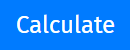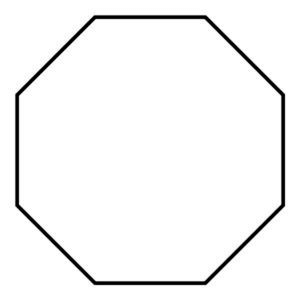# Octagon calculator

Enter Information

#### Result

Fill the calculator form and click on Calculate button to get result here

Get Octagon calculator For Your Website

## Octagon Calculator

Thanks for visiting the octagon calculator. It is an online calculator that lets you calculate the area of an octagon at one click. It only takes some input values to find the area of the octagon. It not only calculates the area of octagon, but also calculates:
•    Sides of octagon
•    Perimeter of octagon
Either you are a student working on your assignment, a teacher checking the work of students, or an engineer, this regular octagon calculator can act as an effective assistant for you.
In this article, we will cover the octagon definition, how to use the octagon calculator, the formula for the area of the octagon, a little bit about octagon sides, and much more. We will also answer some of your questions such as how many sides does an octagon have, how to figure out an octagon, etc.

## How octagon calculator works?

We were so excited while composing this section. The working efficiency of this calculator makes it a reliable and precise tool for calculations. Moreover, the area of an octagon calculator takes ease of use to another level.
You only have to put in the given values in input boxes and bingo!
Follow the below steps to find the area of a regular octagon:
•    Select the value for which you want to make an octagon calculation. i.e., area, perimeter.
•    Select the given set of parameters from the given list.
•    Enter the required value in the input box that appeared below.
•    Hit thebutton to see the result.

### What will you get?

After clicking the Calculate button, it will show you the:
•    Calculated area, perimeter, or side, whatever you selected while filling the form.
•    Formula it used to calculate that value.
•    Step by step calculation of the asked value.
You know that geometrical calculations are always a bit trickier but you don’t need to worry. Apart from the octagon, we have covered the whole geometric range of tools. You can use our triangle area calculator, square calculator, trapezoid area calculator, or hexagon area calculator at any time.

## What is an octagon?

Octagon is an eight-sided shape with eight angles. Octagon shape is a polygon. Below is the standard definition of the octagon by Wikipedia, which states that:
“In geometry, an octagon is an eight-sided polygon or 8-gon. A regular octagon has Schläfli symbol {8} and can also be constructed as a quasiregular truncated square, t{4}, which alternates two types of edges. A truncated octagon, t{8} is a hexadecagon, {16}.”## What are the different types of the octagon?

Octagon has four different types depending on angles and sides.
1.    Regular Octagon: Regular octagon is a standard octagon with eight equal sides and eight equal angles.
2.    Irregular octagon: Irregular octagon is an octagon that has unequal sides and unequal angles.
3.    Convex octagon: If all of the angles of an octagon are pointing outside, it is a convex octagon. Convex octagon has no angles that point inside.
4.    Concave octagon: If one of the angles of an octagon is pointing inwards, it will be a concave octagon.

## Properties of Octagon

The followings are the properties of a regular octagon:
•    Octagon has eight angles and eight sides.
•    All angles and the sides of the octagon are equal.
•    A regular octagon consists of 20 diagonals.
•    Each interior angle in the octagon is equal to 135° and the total sum of the interior angles is 1080°.
•    Each exterior angle in the octagon is equal to 45° and the total sum of the interior angles is 360°.

## Octagon area formula

The formula for area of octagon can be stated as:
A = 2 × (1 + √2) × a2
Where:
A represents the area of the octagon,
a refers to the side of the octagon.

Perimeter of octagon formula

The formula for perimeter of octagon can be written as:
P = a × 8
In this equation, P refers to the perimeter of the octagon, and a refers to the side of the octagon.
Side length of octagon formula
The formula for side length of octagon can be expressed as:
a = P / 8
In this equation, a refers to the side of the octagon, and P refers to the perimeter of octagon.

## How to calculate the area of octagon?

Calculating area of octagon involves working with equations formulas of the octagon. You can use our above octagon calculator to easily find the area of a regular octagon. However, if you want to learn the manual calculation of the octagon area, you are more than welcome.
In this section, we will explain how you can calculate the area of octagon using its formula.
Follow the steps below to find the octagon area:
•    Write down the given values on a paper.
•    Write down the formula of the octagon area.
•    Substitute the values in the octagon area equation and calculate the area.
Let’s understand this topic using an example.

### Example:

Find the area of an octagon having each side of 15 cm?
Solution:
Step 1: Write down the given values on a paper.
a = 15 cm
Step 2: Write down the formula of octagon area.
A = 2 × (1 + √2) × a2
Step 3: Substitute the values in the octagon area equation and calculate the area.
A = 2 × (1 + √2) × 152
A = 2 × 2.4142 × 225
A = 1086.4 cm2

So, an octagon with each side of 15 cm will have an area of 1086.4 cm2.

### Octagon area - Real-world exampleAn octagon-shaped house in Los Angeles has eight octagon-shaped rooms inside it. These rooms cover the complete inner area of the octagon. If each side of the house is 25 feet long, what will be the area of that house?

Solution:
Step 1: Write down the given values.
a = 25 feet
Step 2: Write down the formula of octagon area.
A = 2 × (1 + √2) × a2
Step 3: Substitute the values in the octagon area equation and calculate the area.
A = 2 × (1 + √2) × 252
A = 2 × 2.4142 × 625
A = 3017.75 ft2
So, an octagon-shaped with each side of 25 feet will have an area of 3017.75 ft2.

## FAQs

### What angle is an octagon?

Each interior angle of the octagon is 135° while these interior angles are collectively 1080°. On the other hand, each exterior angle of the octagon is 45° while exterior angles of the octagon are collectively 360°.

### Are all sides of an octagon equal?

Yes, all sides of an octagon are equal in length which means sides of an octagon are congruent. The same goes for the angles of the octagon. All angles of the octagon are the same in size (135°) and accumulate to 1080°.

### How do you find the angles of an octagon?

As we know that all interior angles of an octagon are 1080° jointly. We also know that an octagon has a total of 8 angles of equal side. To calculate the angles of an octagon:
•    Divide 1080° with 8 to get the measurement of each angle.
1080°/8 = 135°
So, each angle in the octagon is 135°.

### Does an octagon have 8 angles?

Yes, an octagon has eight angles in total. Each angle is 135° and all angles add up and make 1080°.

### Does an octagon have right angles?

No, an octagon has no right angles of 90°. That’s because each interior angle of the octagon is 135° while these interior angles are collectively 1080°. On the other hand, each exterior angle of the octagon is 45° while exterior angles of the octagon are collectively 360°.

Other Languages
##### Not Available for Octagon calculator
Related Calculators